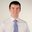Question-and-Answer Resource for the Building Energy Modeling Community
Get started with the Help page

# How to run HVAC system in open-loop (manual air flow rate control)?

I am an electrical engineer trying to develop a thermal model for zone as shown in equation below:

$Tindoor(t+1) = a1Tindoor(t) + a2Toutdoor(t) + a3Qcooling(t) + a4$

where

$Qcooling(t) = <dot>m</dot>air-flowcair(Tsupply - Tindoor)$

To get the constants ($a1, a2, a3, and a4$), I need to keep the $Tsupply$ constant and independently control $<dot>m</dot>air-flow$ i.e. airflow rate set-points to the zones.

By reading the Engineering reference and other answers on this portal, I understand that I have to use

1. AirTerminal:SingleDuct:Uncontrolled terminal for individual zones
2. A constant fan / constant volume zone conditioning component to control the flow and a setpoint manager to control the temperature of the air flowing into the space.

However, I keep getting many errors (such as Thermostat needed for zone even though the terminal is uncontrolled).

Are there any example IDF cases that I can refer or any place where I can get detailed instructions. Thanks.

edit retag close merge delete

Why are you using a constant fan / constant volume zone conditioning component if you want to vary the airflow rate? I would think that you would want a variable speed fan with a constant discharge temperature and a VAV box without a reheat coil.

You need a thermostat in the zone so EnergyPlus knows how to vary the airflow rate (i.e. it needs to know Tindoor(t) like in your equation above).

1

Thanks for the suggestion, I will try with the variable speed fan with constant discharge temperature.

I will add the Thermostat to the zone, but I want to manually control air flow rate. That is why I was using the uncontrolled terminal. Any suggestions on how to manage this issue.

Sort by » oldest newest most votedIf you would like to manually control the air flow rate, I would suggest checking out the suggestions in the following three unmethours posts which uses EMS to manually adjust airflow. I think that's the only way to do what you want.

more